Courses

# Carbonyl Group MCQ - 1 (Advanced)

## 25 Questions MCQ Test Chemistry for JEE Advanced | Carbonyl Group MCQ - 1 (Advanced)

Description
This mock test of Carbonyl Group MCQ - 1 (Advanced) for JEE helps you for every JEE entrance exam. This contains 25 Multiple Choice Questions for JEE Carbonyl Group MCQ - 1 (Advanced) (mcq) to study with solutions a complete question bank. The solved questions answers in this Carbonyl Group MCQ - 1 (Advanced) quiz give you a good mix of easy questions and tough questions. JEE students definitely take this Carbonyl Group MCQ - 1 (Advanced) exercise for a better result in the exam. You can find other Carbonyl Group MCQ - 1 (Advanced) extra questions, long questions & short questions for JEE on EduRev as well by searching above.
QUESTION: 1

Solution:
QUESTION: 2

Solution:
QUESTION: 3

### Which of the following proposed reactions would take place most quickly under mild conditions ?

Solution:
QUESTION: 4

In the given reaction sequene :

C6H5_CN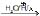A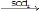B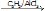C, (C) is

Solution:
QUESTION: 5

H2N–NH2+Ph –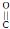–Cl (excess)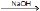X, X is

Solution:
QUESTION: 6

Phosgene(COCl2) + 1 mol C2H5OH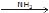X, X is

Solution:
QUESTION: 7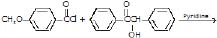X, X is

Solution:
QUESTION: 8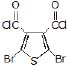+ CH3CH2CH2CH2 NH2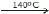X, X is

Solution: The lone pairs of nitrogen will attack at the nucleophilic carbonyl carbon.. 1 mole HCĺ will get eliminated.
again the lone pair of N will attack like previous one and another mole HCl will get eliminated.
in this whole method the carbon chain attached to nitrogen atom will stay unaffected.
hence option c is correct.
QUESTION: 9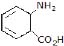+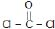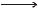X, X is

Solution:
QUESTION: 10

(CH3)3C––Cl+LiAlH4 (excess)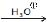X, X is

Solution:
QUESTION: 11

For the given reaction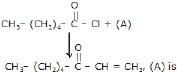Solution:
QUESTION: 12

PhCH2 ––Cl + CH3CH2SH ® X, X is

Solution:
QUESTION: 13

List the following esters in order of decreasing reactivity in the second step of a nucleophilic acyl substitution reaction.

(I)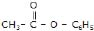(II)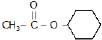(III)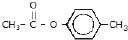(IV)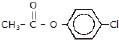Select the correct answer from the codes given below:

Solution:
QUESTION: 14

Which one of the following is least reactive for hydrolysis reaction ?

Solution:
QUESTION: 15

Which one of the following esters is most reactive for saponification ?

Solution:
QUESTION: 16

Consider the following statements for hydrolysis reaction :

(I)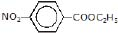is more reactive than C6H5COOC2H5

(II)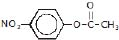is more reactive than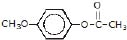(III)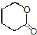is more reactive than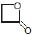Of these the correct statements are

Solution:
QUESTION: 17

Ease of esterification of following acids with CH3OH

(I) HCOOH (II) CH3 COOH (III) CH3 – CH2 – COOH (IV) CH3 –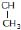– COOH, is

Solution:
QUESTION: 18

The oxidation of primary alcohols give

Solution:
QUESTION: 19

In the given reaction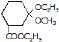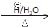[P], [P] will be :

Solution:
QUESTION: 20

In the given reaction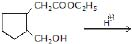[X], [X] is

Solution:
QUESTION: 21

In the given reaction

C2H5–O––O–C2H5+HO–CH 2–CH2–OH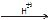[X], [X] is

Solution:
QUESTION: 22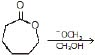X, X is

Solution:
QUESTION: 23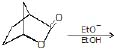Solution:
QUESTION: 24

Ease of esterification of following alcohol with HCOOH is

(I) CH3–CH2OH (II) (CH3)2CH–OH (III) (CH3)3C–OH

Solution:
QUESTION: 25

The product x in the above reaction is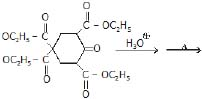Solution: